GeoA - Chapter 1 Skills Maint 1
Identify the definition of bisector *
1 point
Can two non adjacent angles be complementary and supplementary at the same time? *
1 point
What is the measure of an angle complementary to an angle with a measure of 54 degrees? *
1 point
Last Name *
Find x if m is the midpoint of segment DE *
1 point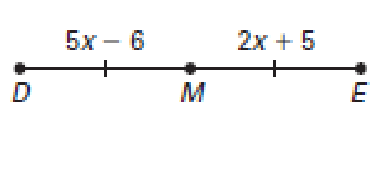What geometric figure is this? *
1 point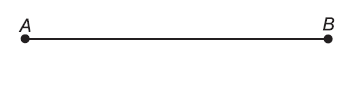S is the midpoint of segment RT. Find x. *
1 point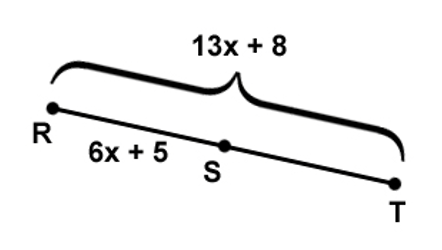These two angles are..... *
1 point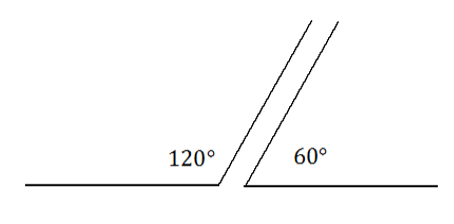Find angle z only *
1 point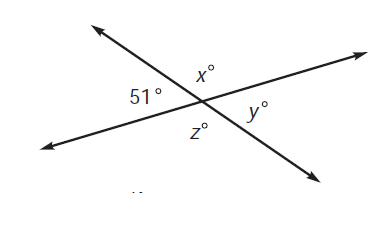If CD = 5x - 7, Find x. *
1 point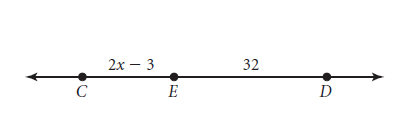Period *
Two angles that add up to 180 degrees are... *
1 point
Angles 1 and 3 are.... *
1 point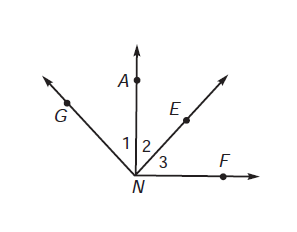Find x *
1 point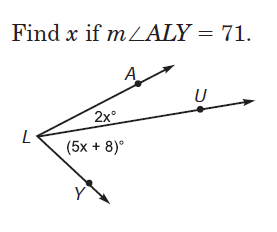Find x *
1 point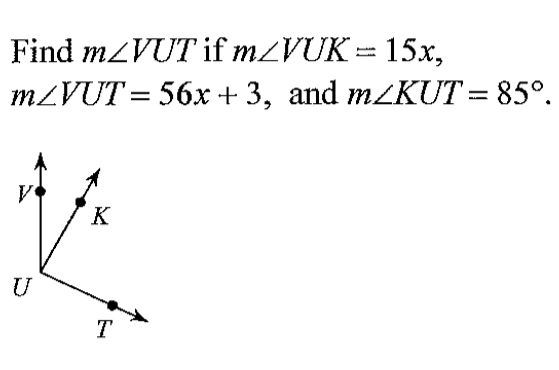Which option is the INCORRECT way to name this picture? *
1 point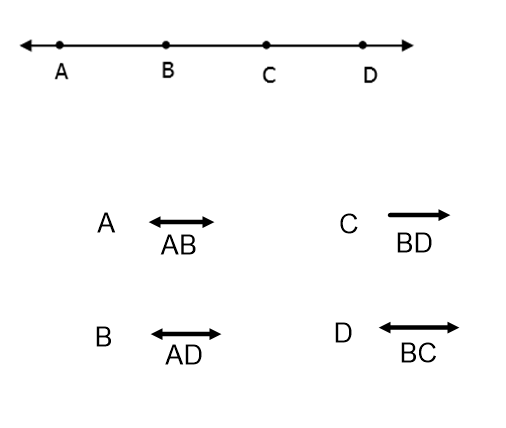Find the measure of angle 2 *
1 pointCan two non adjacent angles be complementary? *
1 point